# Fluid Mechanics and Thermal Sciences - Online Test

Q1. A solid sphere of radius r1 = 20 mm is placed concentrically inside a hollow sphere of radius r2 = 30 mm as shown in the figure.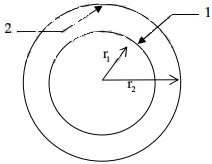The view factor F21 for radiation heat transfer is
Explaination / Solution: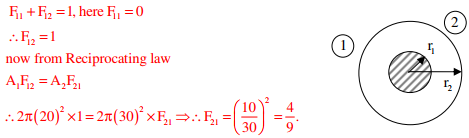Q2. If a mass of moist air in an airtight vessel is heated to a higher temperature, then
Explaination / Solution:
No Explaination.

Q3. In a condenser of a power plant, the steam condenses at a temperature of 60o C . The cooling water enters at 30o C and leaves at 45o C . The logarithmic mean temperature difference (LMTD) of the condenser is
Explaination / Solution:

Flow configuration in condenser as shown below.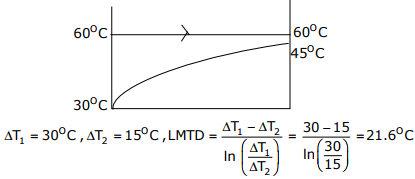Q4. Heat and work are
Explaination / Solution:

Heat and work are path functions. Since δQ and δW are dependent on path followed between two given end states of a thermodynamic process undergone by system.

Q5. A pipe of 25mm outer diameter carries steam. The heat transfer coefficient between the cylinder and surroundings is 5W /m2K . It is proposed to reduce the heat loss from the pipe by adding insulation having a thermal conductivity of 0.05W/mK. Which one of the following statements is TRUE?
Explaination / Solution:
No Explaination.

Q6. The contents of a well-insulated tank are heated by a resistor of 23Ω in which 10A current is flowing. Consider the tank along with its contents as a thermodynamic system. The work done by the system and the heat transfer to the system are positive. The rates of heat (Q), work (W) and change in internal energy (∆U) during the process in kW are
Explaination / Solution: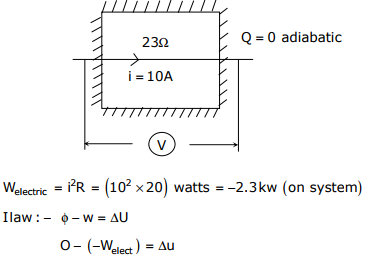Q7. Figure shows the schematic for the measurement of velocity of air (density = 1.2kg /m3 ) through a constant–area duct using a pitot tube and a water-tube manometer. The differential head of water (density = 1000 kg /m3) in the two columns of the manometer is 10mm. Take acceleration due to gravity as 9.8m / s2. The velocity of air in m/s isExplaination / Solution:

From Bernoulli’s equation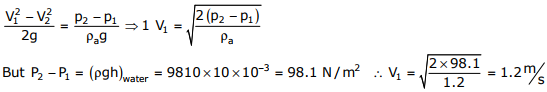Q8. The values of enthalpy of steam at the inlet and outlet of a steam turbine in a Rankine cycle are 2800kJ/kg and 1800kJ/kg respectively. Neglecting pump work, the specific steam consumption in kg/kW-hour is
Explaination / Solution:

Work done by the turbine
W = 2800 – 1800 = 1000 kJ/kg = 1000 kW-s/kg
Specific fuel consumption = (1/1000)×3600 = 3.6 kg / kw-hr

Q9. The ratios of the laminar hydrodynamic boundary layer thickness to thermal boundary layer thickness of flows of two fluids P and Q on a flat plate are 1/2 and 2 respectively. The Reynolds number based on the plate length for both the flows is 104. The Prandtl and Nusselt numbers for P are 1/8 and 35 respectively. The Prandtl and Nusselt numbers for Q are respectively
Explaination / Solution: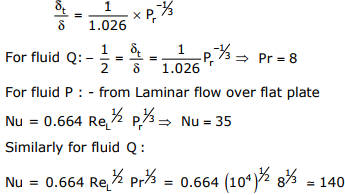Q10. The crank radius of a single–cylinder I. C. engine is 60mm and the diameter of the cylinder is 80mm. The swept volume of the cylinder in cm3 is
Explaination / Solution:

Stroke of the cylinder l = 2r = 2 × 60 = 120mm
Swept volume = (π/4)d2 × I = (π/4) × 802 × 120 = 603 cm3